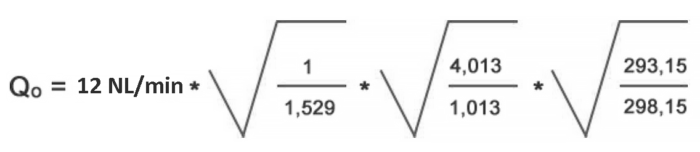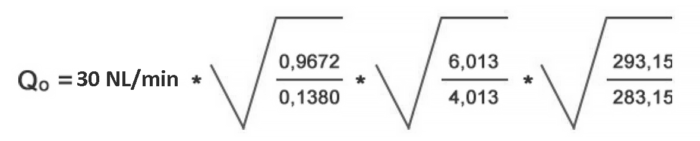Country & language: Canada / English# Gas flow meter calculation formulas

Here you can find calculation formulas for gas flow conversions. Use the conversion formula shown below. Here’s also few examples to help you!

### Gas flow conversion formula### Actual flow rate

Q0 = Actual flow rate

ρ0 = Specific gravity of measured gas (Air = 1)

p0 = Actual ABSOLUTE pressure in metering tube

T0 = Actual ABSOLUTE temperature
(Kelvin) in metering tube

(0°C = 273,15°K)
(20° C = 293,15°K)

### Flowmeter design (calibration) conditions

ρ1 = Specific gravity of design gas (Air = 1)

p1 = ABSOLUTE design pressure
(calibration pressure)

T1 = ABSOLUTE design temperature
(Calibration temperature)

## Example 1

What is the maximum flow if flow meter EK-3NR is used for carbon dioxide at 3 bar(g) / 25°C.

Ex-3NR scale is

• 1 – 12 NL/min air at 0 bar(g)/20°C

Q1 = 12 NL/min
ρ1 = 1
p1 = (0+1,013) bar = 1,013 bar
T1 = 293,15°K

Q0 = ?
ρ0 = 1,529
p0 = (3+1,013) bar = 4,013 bar
T0 = 298,15°KActual flow at 12 NL/min reading
Q0 = 19,15 NL/min CO2 at 3 bar(g)/ 25°C

## Example 2

Flow meter LH-JJ32 is calibrated for 30 NL/min nitrogen at 3 bar(g)/ 20°C

What is the actual flow if flow meter LH-JJ32 is used for helium at 5 bar(g) / 10°C.

Q1 = 30 NL/min
ρ1 = 0,9672
p1 = (3+1,013) bar = 4,013 bar
T1 = 293,15°K

Q0 = ?
ρ0 = 0,1380
p0 = (5+1,013) bar = 6,013 bar
T0= 283,15°KActual flow at 30 NL/min reading
Q0 = 98,9 NL/min He at 5 bar(g)/ 10°C

### Let's get in touch

Kytola Instruments Ltd.
1321 Blanshard Street, Suite 301
Victoria, BC V8W 0B6

Tel: +1-678-701-3569 (English)
Tel: +1-514-691-9945 (French)
Fax: +1-514-448-5151

Orders and General Email: flow@kytola.ca

Accounting: accounting@kytola.ca

Hidden
Hidden
This field is for validation purposes and should be left unchanged.

#### Sales

gas-flow-meter-calculation-formulas

gas-flow-meter-calculation-formulas

#### President

gas-flow-meter-calculation-formulas